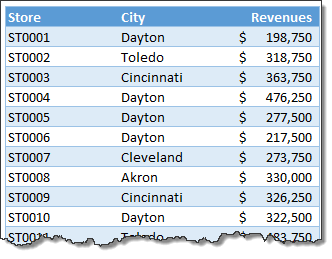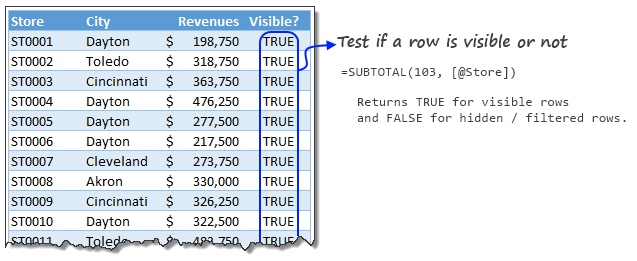# Calculate sum of top 10 values [formulas + homework]

Share

Analyzing top n (or bottom m) items is an important part of any data analysis exercise. In this article, we are going to learn Excel formulas to help you with that.

Let’s say you are the lead analyst at a large retail chain in Ohio, USA. You are looking at the latest sales data for all the 300 stores. You want to calculate the total sales of top 10 stores.  Read on to learn the techniques.

### Meet the data

So here is the data we have. It is arranged in an Excel table, named Sales.We need to answer to 2 questions.

• What is the sum of top n sales?
• What is the sum of top n sales for filtered data (say store=Dayton)?

### Sum of top n sales

First let’s take a look the formula.

`=SUMIFS(sales[Revenues],sales[Revenues],">="&LARGE(sales[Revenues],n))`

[Related: using structural references in Excel]

How does this formula work?

There are 2 components in this formula:

1. We need to sum up revenues column
2. Such that, revenue >= top nth revenue

Finding the top nth value:

This is where LARGE formula helps. It looks at the revenue column and returns nth value.

Sum of top n values thru SUMIFS:

Then, SUMIFS formula calculates the total revenues where revenue >= top nth value.

[Related: Introduction to SUMIFS formula]

### Sum of top n sales in filtered data

This one is tricky. First, we will add an extra column to the sales table. You can later hide this if you want.

This column just tells us whether a particular store is hidden or visible (ie filtered away or not).

Use the formula,

`=SUBTOTAL(3, [@Store]) = 1` in the new column. This will be TRUE if a row is visible and FALSE if a row is filtered away.

See below illustration to understand the formula.Next, we can use below formula to calculate the total of top n sales in filtered data:

`=SUMIFS(sales[Revenues],sales[Visible?],TRUE, sales[Revenues],">="&AGGREGATE(14,5,sales[Revenues],n))`

How does this formula work?

Again, we are using SUMIFS formula, but with 2 conditions.

1. Store should be visible
2. Revenue >= top nth revenue in visible stores

To calculate the top n value of a visible stores, we use AGGREGATE formula.

`AGGREGATE(14,5,sales[Revenues],n)` – what does it do?

AGGREGATE formula takes 3 or 4 parameters.

1. Calculation number – 14 corresponds to LARGE
2. Which data to ignore – 5 corresponds to ‘ignore hidden rows’
3. Data – Sales[Revenues]
4. n – optional parameter for LARGE or SMALL calculations

So, our `AGGREGATE(14,5,sales[Revenues],n)` formula will return top nth value among the filtered data.

Once we know that value, we just use SUMIFS to sum up all values greater than or equal to it.

### Your home work – 2 challenges:

So now that you understood how to calculate sum of top n values, I have 2 home work problems.

1. What is the sum of bottom 10 values excluding zero values?
2. What is the sum of bottom 10 values in filtered list, excluding zeros?

### 6 more tips on analyzing top n values

Here are few more ways to analyze with top /bottom n  values.

This post is part of our Awesome August  Excel Festival.

### Get FREE Excel + Power BI Tips

Simple, fun and useful emails, once per week.

Learn & be awesome.

### Welcome to Chandoo.org

Thank you so much for visiting. My aim is to make you awesome in Excel & Power BI. I do this by sharing videos, tips, examples and downloads on this website. There are more than 1,000 pages with all things Excel, Power BI, Dashboards & VBA here. Go ahead and spend few minutes to be AWESOME.

Read my storyFREE Excel tips book

I will be presenting at two events in London in April 2020. Come & join me.

Chandoo is an awesome teacher
5/5

– Jason

Still on fence about Power BI? In this getting started guide, learn what is Power BI, how to get it and how to create your first report from scratch.

### 35 Responses to “Calculate sum of top 10 values [formulas + homework]”

1.Chandra MOhan says:

1.the sum of bottom 10 values excluding zero values = {=SUMIFS(sales[Revenues],sales[Revenues],"0,sales[Revenues]),10))}

2. the sum of bottom 10 values in filtered list, excluding zeros = {=SUMIFS(sales[Revenues],sales[Visible?],TRUE,sales[Revenues],"0,sales[Revenues]),10))}

•Chandra MOhan says:

complete formula not pasted

for condtion 1 {=SUMIFS(sales[Revenues],sales[Revenues],"0,sales[Revenues]),10))}

for condition 2 {=SUMIFS(sales[Revenues],sales[Visible?],TRUE,sales[Revenues],"0,sales[Revenues]),10))}

•chandra mohan says:

@chandoo , why full formula not pasting here??

2.Ashish says:

Condition 1:
=SUMIFS(sales[Revenues]|sales[Revenues]|"<="&SMALL(sales[Revenues]|COUNTIF(sales[Revenues]|0)+10))

Condition 2:
=SUMIFS(sales[Revenues]|sales[Visible?]|TRUE|sales[Revenues]|"<="&SMALL(sales[Revenues]|COUNTIF(sales[Revenues]|0)+10))

3.cinnabar says:

Awesome post !! The subtotal trick can come in very handy for checking data hidden by table filters.

4.Uriel says:

Hi all, I use excel 2007 so AGGREGATE doesn't works. How can I substitute the AGGREGATE function in this formula: "=SUMIFS(sales[Revenues],sales[Visible?],TRUE,sales[Revenues],">="&AGGREGATE(14,5,sales[Revenues],n))?
in other words, How can I make that LARGE ignore hidden rows?.

Regards

•NARAYAN says:

Hi ,

See if this works :

=SUMIFS(sales[Revenues],sales[Visible?],TRUE,sales[Revenues],">="&LARGE(IF(sales[Visible?],sales[Revenues]),n))

entered as an array formula , using CTRL SHIFT ENTER.

•Elias says:

Change formula of Visible? field to
=SUBTOTAL( 103 , [@Store] ) * [@Revenues]

Then change the main formula to
=SUMIFS( sales[Visible?] , sales[Visible?] , " > = " & LARGE( sales[Visible?] , n ) )

Regards

5.The Stig says:

Hi Chandoo. I have a question about this method. Does this method stand up if you have potential duplicate values in the Top 10. For example, if you are scoring a sporting event where competitors earn a fixed score based on their performance. For example auto racing. There are 15 events however only the Top 10 count towards the final standings.

What are the Top 10 scores for this participant?

Final Scores After 15 Events:
200
200
175
155
175
140
130
140
175
200
130
120
110
200
155

6.Leonid says:

Because we might have ties in the dataset it's more accurate to use
=SUMPRODUCT(LARGE(sale[Revenues],ROW(\$A\$1:INDEX(A:A,5,))))

•The Stig says:

Thanks Leonid. That works. Can you explain the basics of this formula? I tried to break it apart to see how it works but don't see all the connections between the formula's.

•Leonid says:

@Stig,
The formula for top N numbers that I provided: =SUMPRODUCT(LARGE(sale[Revenues],ROW(\$A\$1:INDEX(A:A,N,))))
and the formula @jason m provided: =SUMPRODUCT(LARGE(range,ROW(INDIRECT(“1:N”)))) are logicaly the same.
The portion with ROW function generates an array of sequential numbers from 1 to N: {1;2;..N}
LARGE function receives this array as a parameter, calculates the value for each item in the {1;2;..N} array and pass the array of results to SUMPRODUCT function to sum them up.

•The Stig says:

Thanks for the explanation. What does the INDEX function do inside the ROW function?

•Leonid says:

INDEX(A:A,N,)return the cell in column A:A in row N.
E.g. INDEX(A:A,10,) is A10. Glued with A1 by range operator ":" it gives us a range A1:A10.
Then ROW(A1:A10) returns an array of sequential numbers from 1 to 10.

•feldspath says:

For the question asked by "The Stig" the number in the formula given by Leonid must be 10 instead of 5, since the top 10 scores must be counted:

=SUMPRODUCT(LARGE(sale[Revenues],ROW(\$A\$1:INDEX(A:A,10,))))

•Blaz says:

hey Leonid,

can you explain the steps in the formula and why it works?

thanks.

7.MF says:

Answer 1 (since you have no negative number, a simply trick would do)
=SUMIF(sales[Revenues],"<="&SMALL(sales[Revenues],10+COUNTIF(sales[Revenues],0)))

Answer 2 (Obviously, we need Excel 2010 or later)
=SUMPRODUCT(AGGREGATE(15,6,1/(1/(sales[Revenues]*sales[Visible?])),ROW(A1:A10)))

•MF says:

Side topic:
Pivot Table should be the easiest way to find the answer. But I can understand that it's a demonstration of a formula approach. 🙂

8.Peahead says:

Here are the formula's I came up with to solve the 2 challenges:

1 - =SUMIFS(sales[Revenues],sales[Revenues],"<="&SMALL(sales[Revenues],n+COUNTIF(sales[Revenues],0)))

2 - =SUMIFS(sales[Revenues],sales[Visible?],TRUE,sales[Revenues],"<="&AGGREGATE(15,5,sales[Revenues],n+COUNTIFS(sales[Visible?],TRUE,sales[Revenues],0)))

9.handsome says:

I adopted a somewhat different approach. I added a new column (F). This column was populated with the following formula:
=IF([@Revenues]>0,RANK([@Revenues],[Revenues],0),"")

The formula I used for Question 1 was as follows:
=SUMIF(sales[Rank],">"&MAX(sales[Rank])-n,sales[Revenues])

The formula I used for Question 2 was as follows:
=SUMIFS(sales[Revenues],sales[Rank],">"&MAX(sales[Rank])-n,sales[Visible?],TRUE)

Hopefully that's correct!

10.jason m says:

SUMIFS is for millenials. 😉

Top 10
=SUMPRODUCT(LARGE(Sales[Revenues],ROW(INDIRECT("1:10"))))

Bottom 10
=SUMPRODUCT(SMALL(Sales[Revenues],ROW(INDIRECT("1:10"))))

•The Stig says:

Hi Jason. How would this formula work if the number go across a row vs. down a column?

200 200 175 155 175 140 130 140 175 200 130 120 110 200 155

•jason m says:

Yes. The basic formula to sum the top N numbers is:

=SUMPRODUCT(LARGE(range,ROW(INDIRECT("1:N"))))

where "range" is the group of contiguous cells.

•The Stig says:

Cool, thanks. I have one more question... I "have a friend" that uses Numbers for Mac. Do you happen to know if the formula works in Numbers?

•MF says:

Bottom 10
=SUMPRODUCT(SMALL(Sales[Revenues],ROW(INDIRECT(“1:10?))))

Hi Jason, this doesn't exclude zero value as requested.

•jason m says:

Array-entered:

=SUM(SMALL(IF(Sales[Revenues]>0,Sales[Revenues]),ROW(INDIRECT("1:10"))))

11.Leonid says:

This array formula
={SUMPRODUCT(IFERROR(SMALL(MODE.MULT(IF(sales[Revenues]0,{1,1}*sales[Revenues])),ROW(\$A\$1:INDEX(\$A:\$A,MIN(SUM(N(sales[Revenues]0)),10)))),0))}
returns sum of the bottom 10 values excluding zero values and also ignore ties and handles the case when we have less than 10 values to sum up or when all values are 0s.

•Leonid says:

={SUMPRODUCT(IFERROR(SMALL(MODE.MULT(IF(sales[Revenues]<>0,{1,1}*sales[Revenues])),ROW(\$A\$1:INDEX(\$A:\$A,MIN(SUM(N(sales[Revenues]<>0)),10)))),0))}

•Leonid says:

=SUMPRODUCT(IFERROR(SMALL(IFERROR(sales[Revenues]/(sales[Revenues]<>0),""),ROW(A1:INDEX(A:A,MIN(SUM(N(sales[Revenues]<>0)),10),))),0))

12.Andreia says:

What if I wanted to sum the top 10 sales of each store from a sheet that has the sales of all stores?

13.Muhammad Ramzan says:

I am trying to sum of "credit" against top 5 "Revenue" customers using this array formula:
{=SUM(IF(LARGE(Sales[Revenue],{1,2,3,4,5}),1,0)*Sales[Credit])}
But it is giving sumproduct of all the value !

Please correct this formula or suggest any other solution.

•Chandoo says:

Try this...

{=SUM(IF(LARGE(Sales[Revenue],{1,2,3,4,5})=Sales[Revenue],1,0)*Sales[Credit])}

•Muhammad Ramzan says:

Thanks Chandoo, given solution is working fine.

14.Alper says:

For top of 10 values with filtered data with excluding zero and negative values;

=SUMPRODUCT(SMALL(IF(--sales[Visible?]*sales[Revenues]>0;sales[Revenues];" ");{1;2;3;4;5;6;7;8;9;10}))

Explanation:

--sales[Visible?]*sales[Revenues]>0 this part eliminated the invisible cells and also negative and zero revenues;

if it is invisible it will be 0 in visible side thus product will be 0,and it can be said the same for zero revenues

if it is negative in revenue side the product will be negative; thus they are eliminated.

and finally, extracting smallest 10 values rest of the values, which are visible, nonnegative and nonzero, and their sum.

•Alper says:

=SUMPRODUCT(SMALL(IF(--sales[Visible?]*sales[Revenues]>0,sales[Revenues]," "),{1,2,3,4,5,6,7,8,9,10}))

It must be entered as an ARRAY formula.

### Get FREE Excel & Power-BI Newsletter

One email per week with Excel and Power BI goodness. Join 100,000+ others and get it free.